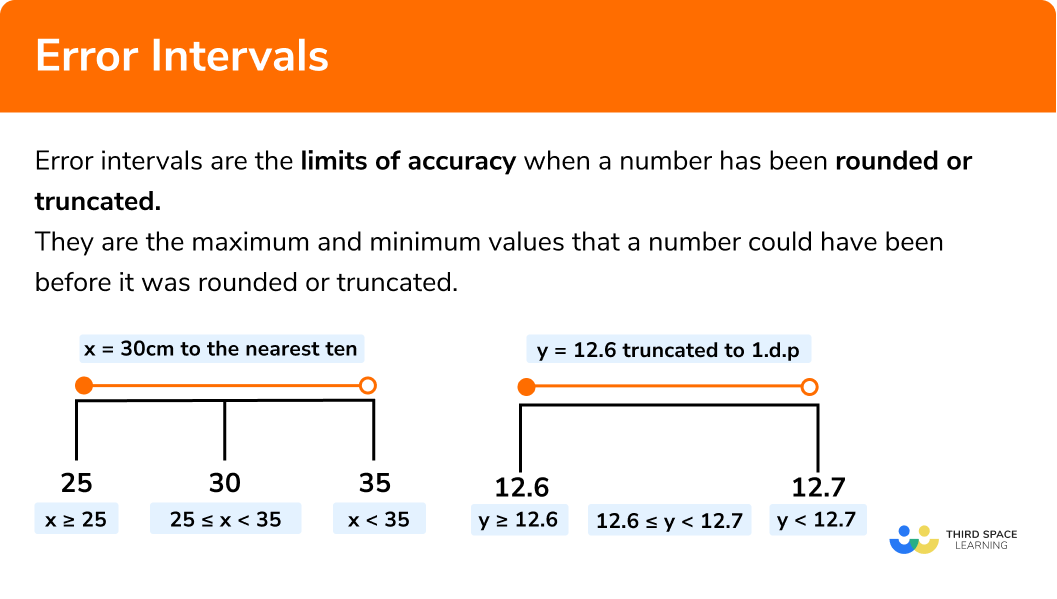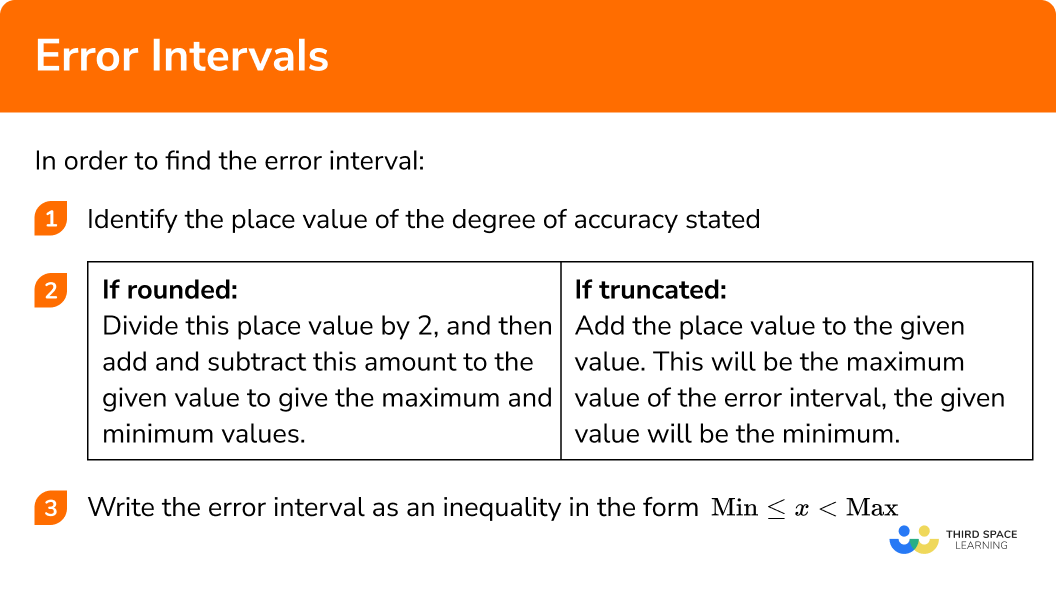# Error Intervals

Here we will learn about error intervals including how to find error intervals for numbers that have been rounded and for truncated numbers.

There are also error intervals worksheets based on Edexcel, AQA and OCR exam questions, along with further guidance on where to go next if you’re still stuck.

## What are error intervals?

Error intervals are the limits of accuracy when a number has been rounded or truncated. They are the range of possible values that a number could have been before it was rounded or truncated.

To do this we think about what the smallest and biggest numbers that would round, or be truncated, to a value for a given degree of accuracy.

E.g.

### What are error intervals?## How to find error intervals

In order to find the error interval of a rounded or truncated number:

1. Identify the place value of the degree of accuracy stated – this will be the interval size required for the error interval.

2

If rounded:

Divide this place value by 2, and then add and subtract this amount to the given value to give the maximum and minimum values for your error interval

If truncated:

Add the place value to the given value.
This will be the maximum value of your error interval, the given value

3 Write your error interval as an inequality in the form Min   ≤ x <   Max

For the case of rounded numbers, the maximum and minimum are referred to as the upper bound and lower bound of the number.

### Explain how to find error intervals in 3 steps## Error interval examples

### Example 1: finding the error intervals of numbers rounded to the nearest whole number

The height of a house, h, was measured as 7 metres to the nearest metre.
Find the error interval of the height of the house.

1. Identify the place value of the degree of accuracy stated – this will be the interval size required for the error interval.

The place value of the degree of accuracy is 1.

2 If rounded: Divide this place value by 2, and then add and subtract this amount to the given value to give the maximum and minimum values for your error interval.

$1\div 2=0.5$

Maximum = 7 + 0.5 = 7.5 m
Minimum = 7- 0.5 = 6.5 m

3 Write your error interval as an inequality in the form Min  ≤ x <   Max

6.5 m   ≤ h <   7.5 m

### Example 2: finding the error intervals of numbers rounded to the nearest 10,100,1000,…

The mass of a stone, m, was measured as 400g to the nearest 100g. Find the error interval of the mass of the stone.

The place value of the degree of accuracy is 100.

$100\div 2=50$

Maximum = 400 + 50 = 450g
Minimum = 400 – 50 = 350g

350g   ≤ m <   450g

### Example 3: finding the error intervals of numbers rounded to decimal places

The length of a pencil, l,  was measured as 22.7cm to the nearest mm. Find the error interval of the length of the pencil.

1mm = 0.1cm.

The place value of the degree of accuracy is 0.1.

$0.1\div 2=0.05$

Maximum = 22.7 + 0.05 = 22.75 cm

Minimum = 22.7 – 0.05 = 22.65 cm.

22.65 cm   ≤ l <   22.75 cm

### Example 4: finding the error intervals of numbers rounded to significant figures

The attendance, a, to a rugby match was recorded as 38000 people rounded to 2 significant figures. Find the error interval of the attendance to the rugby match.

The second significant figure is the 8, which represents 8 thousand.
The place value of the degree of accuracy is 1000.

$1000\div 2=500$

Maximum =  38000 + 500 = 38500 people

Minimum = 38000 – 500 = 37500 people

37500   ≤ a <   38500

### Example 5: finding the error intervals of truncated numbers

When asked her age, Imogen said that she was 13 years old. Imogen truncated her age to the integer value. Find the error interval for Imogen’s age, x

The place value of the degree of accuracy is 1

$13 + 1 = 14$

Maximum = 14

Minimum = 13

13   ≤ x <   14

### Example 6: finding the error intervals of truncated numbers

Sam calculated the area of a circle and truncated the answer to 2 decimal places. His answer was 49.26 cm2. Find the error interval for the area of the circle, A.

The place value of the degree of accuracy is 0.01

$49.26 + 0.01 = 49.27$

Maximum = 49.27

Minimum = 49.26

49.26 cm2   ≤ A <   49.27 cm2

### Common misconceptions

• Maximum value is incorrect

It is very common for students to put an incorrect maximum value,

E.g

If a length has been rounded to 16 cm to the nearest centimetre, they may incorrectly write the error interval as 15.5 cm   ≤ x <   16.4 cm.

The maximum value is given using a strict inequality.
x could have been 16.41cm or 16.49 cm or 16.499 cm or 16.499999…, so the important thing is that it must be less than 16.5 cm.

• Incorrect use of inequality notation

It is important that the correct inequality symbols are used in the error interval. The range of values must be greater than or equal to the minimum, and strictly less than the maximum.

• The wrong methods are used for rounded or truncated numbers

It is important to read the questions very carefully and look out for the word “truncated”. The exam questions will not always use the word “rounded” in a question.

E.g.
“The length y was written to the nearest 10 kilometres”. If the word “nearest” is used, you know the number was rounded, if the number has been truncated it will be stated clearly.

Error intervals are part of our series of lessons to support revision on rounding numbers, and upper and lower bounds. You may find it helpful to start with the main rounding numbers lesson for a summary of what to expect, or use the step by step guides below for further detail on individual topics. Other lessons in this series include:

### Practice error interval questions

1. The mass, m , of a rock is 24 kilograms to the nearest kilogram.

Complete the error interval for the mass of the rock.

….. ≤ m < ……

24 kg ≤ m < 25 kg24 kg ≤ m < 24.5 kg23.5 kg ≤ m < 24.5 kg23.5 kg ≤ m < 24.49 kgThe mass is rounded to the nearest integer (the ones column). Divide this place value by 2 , and then add and subtract this amount to the given value to give the maximum and minimum values for your error interval.

2. Jonny estimates the number of steps, s , he has walked in one day to be 7000 to the nearest 1000 .

Complete the error interval for Jonny’s estimate.

….. ≤ s < ……

6000 ≤ s < 75006000 ≤ s < 70006500 ≤ s < 75006999 ≤ s < 7500The number of steps is rounded to the nearest 1000 . Divide this place value by 2 , and then add and subtract this amount to the given value to give the maximum and minimum values for your error interval.

3. The circumference, C , of a circle is 63.5 cm , rounded to three significant figures.

Complete the error interval for the circumference of the circle.

….. ≤ C < ……

63.4 cm ≤ C < 63.5 cm63.49 cm ≤ C < 63.59 cm63.45 cm ≤ C < 63.55 cm63 cm ≤ C < 64 cmThe circumference is rounded to three significant figures, which takes us up to the tenths. Divide this place value by 2 , and then add and subtract this amount to the given value to give the maximum and minimum values for your error interval.

4. The length, L , of a river is 5700 kilometres, truncated to two significant figures.

Find the error interval of the length of the river.

5600 km ≤ L < 5800 km5650 km ≤ L < 5750 km5700 km ≤ L < 5800 km5650 km ≤ L < 5800 kmThe length is truncated to two significant figures, which is in the hundreds column. Add this place value to the given value. This will be the maximum value of your error interval, the given value will be your minimum.

5. The age, A , of a fossil is 34,000,000 years to the nearest million.

Find the error interval for the age of the fossil.

33,000,000 years ≤ A < 34,000,000 years33,500,000 years ≤ A < 34,500,000 years33,550,000 years ≤ A < 34,550,000 years34,000,000 years ≤ A < 35,000,000 yearsThe age is rounded to the nearest 1,000,000 . Divide this place value by 2 , and then add and subtract this amount to the given value to give the maximum and minimum values for your error interval.

6. An average speed calculation, S , is 93.2 km/h truncated to one decimal place.

Find the error interval for S .

93.25 km/h ≤ S < 93.35 km/h93.15 km/h ≤ S < 93.35 km/h93 km/h ≤ S < 94 km/h93.2 km/h ≤ S < 93.3 km/hThe speed is truncated to the tenths. Add this place value to the given value. This will be the maximum value of your error interval, the given value will be your minimum.

### Error interval GCSE questions

1. Kevin truncates the number x to one decimal place. The result is 8.4 .

Write the error interval for x .

(2 marks)

8.4   or   8.5

(1)

8.4  ≤ x <   8.5

(1)

2. Ana rounds the number y to 2 significant figures. The result is 7200 .

Write the error interval for y .

(2 marks)

7150  or  7250

(1)

7150  ≤ y <   7250

(1)

3. Amir measures a door to be 782mm wide to the nearest mm .

Write the error interval for the width of the door w .

(2 marks)

781.5   or   782.5

(2)

781.5 mm  ≤ w <   782.5 mm

(2)

## Learning checklist

You have now learned how to:

• Apply and interpret limits of accuracy when rounding or truncating

## Still stuck?

Prepare your KS4 students for maths GCSEs success with Third Space Learning. Weekly online one to one GCSE maths revision lessons delivered by expert maths tutors.

Find out more about our GCSE maths tuition programme.